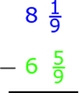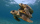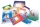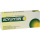# Estimate

Estimate the answer by rounding each fraction to the nearest whole number and then subtracting. 9/15 - 2 7/8

e =  -2
x =  -2.275

### Step-by-step explanation:Did you find an error or inaccuracy? Feel free to write us. Thank you!Tips to related online calculators
Need help to calculate sum, simplify or multiply fractions? Try our fraction calculator.
Do you want to round the number?

## Related math problems and questions:

• To improper fractionChange mixed number to improper fraction a) 1 2/15 b) -2 15/17
• Evaluate 17Evaluate 2x+6y when x=- 4/5 and y=1/3. Write your answer as a fraction or mixed number in simplest form.
• The sum 12The sum of 3 mixed numbers is 20 13/15. two of the numbers are 6 1/3 and 7 5/6. what is the third number?
• 15 numberWhat number is smaller (greater) by 15 than its half?
• Divide 11Divide the product of 4 and 5/8 by 1 1/2. Write your answer as a mixed number.
• Sum of mixedThe sum of two mixed numbers is 15½. One of the number is 5 ¾, what is the other?
• A turtleA turtle is 20 5/6 inches below the surface of a pond. It dives to a depth of 32 1/4 inches. What is the change in the turtle’s position? Write your answer as a mixed number.
• How manyHow many integers are greater than 547/3 and less than 931/4?
• Fractions and mixed numerals(a) Convert the following mixed numbers to improper fractions. i. 3 5/8 ii. 7 7/6 (b) Convert the following improper fraction to a mixed number. i. 13/4 ii. 78/5 (c) Simplify these fractions to their lowest terms. i. 36/42 ii. 27/45 2. evaluate the follow
• Mixed numbersRewrite mixed numbers, so the fractions have the same denominator: 5 1/5 - 2 2/3
• JonahJonah has an 8-pound bag of potting soil. He divides it evenly among 5 flowerpots. How much soil is in each pot? Show your answer as a fraction or mixed number. Solve this problem any way you choose.
• A 14.5-gallonA 14.5-gallon gasoline tank is 3/4 full. How many gallons will it take to fill the tank? Write your answer as a mixed number.
• Each yearEach year, Mr. Baumann purchases enough paper for his 16 students. If each student will use 3/4 pack of paper, how many packs of paper will he need to purchase? Answer as a mixed number or as a whole number.
• Simplest form of a fractionWhich one of the following fraction after reducing in simplest form is not equal to 3/2? a) 15/20 b) 12/8 c) 27/18 d) 6/4
• BrianBrian divides 80 by 7. Between what two whole numbers is his answer?
• RegroupingSubtract mixed number with regrouping: 11 17/20- 6 19/20
• Fraction of a NumberIf 1/2 of 1/3 of 1/4 of 1/5 of a number is 2.5. what is the number?